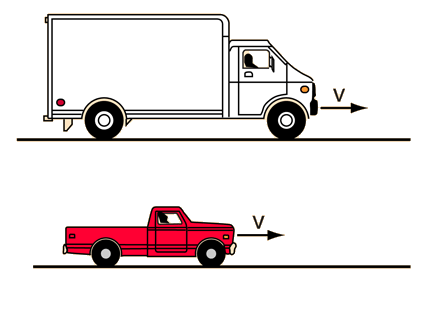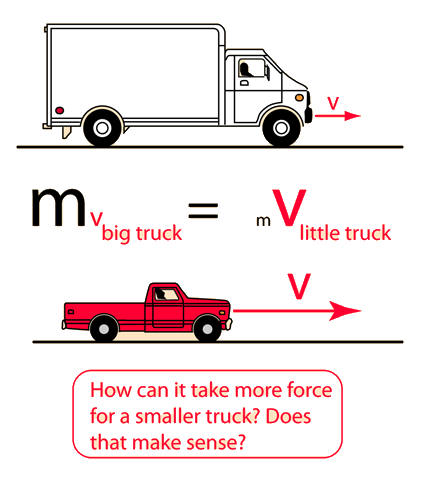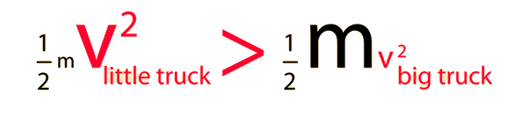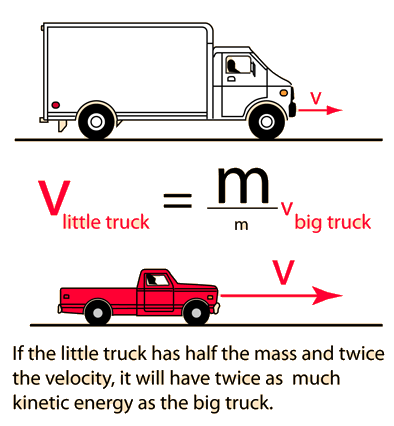# Stopping a TruckSuppose a large truck and a small truck are moving with equal momentum. Which will require more work to stop? Which will require more force to stop in a given distance?

### Discussion of the questions

Index

Collision concepts

 HyperPhysics***** Mechanics ***** Newton's laws R Nave
Go Back

# More force to stop a smaller truck?With equal momentum, the little truck will have more kinetic energy since kinetic energy depends upon the square of the velocity. With more kinetic energy, it will take more work to stop the smaller truck. Since work is equal to average force times stopping distance, the work-energy principle implies that it will take more force to stop the smaller truck in a given distance.Index

Collision concepts

 HyperPhysics***** Mechanics ***** Newton's laws R Nave
Go Back

# How can it take more force to stop a smaller truck?If the big truck and the smaller truck have equal momentum, as stated in the equal momentum example, then the smaller truck will have to have a higher velocity. Since kinetic energy depends upon the square of the velocity, this implies that the smaller truck will have a higher kinetic energy. By the work-energy principle, then, it will take more work to stop the smaller truck.

If the trucks were traveling at the same speed, it would of course take more work to stop the bigger truck.

### Collision questions

Index

Collision concepts

 HyperPhysics***** Mechanics ***** Newton's laws R Nave
Go Back